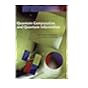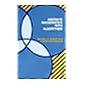Normal view MARC view ISBD view

# Quantum computation and quantum information / Michael A. Nielsen and Isaac L. Chuang.

Material type:TextPublisher: Cambridge ; New York : Cambridge University Press, 2000Description: xxv, 676 p. : ill. ; 26 cm.ISBN: 0521632358; 0521635039 (pbk.); 9780521632355.DDC classification: 511.8
Contents:
Table of contents Fundamental Concepts -- Introduction and overview -- Introduction to quantum mechanics -- Introduction to computer science -- Quantum Computation -- Quantum circuits -- The quantum Fourier transform and its applications -- Quantum search algorithms -- Quantum computers: physical realisation -- Quantum Information -- Quantum noise, open quantum systems, and quantum operations -- Distance measurement for quantum information -- Quantum error-correction -- Entropy and information -- Quantum information theory -- Notes on basic probability theory -- Group theory -- Approximating quantum gates: the Solovay-Kitaev theorem -- Number theory -- Public-key cryptography and the RSA cryptosystem -- Proof of Lieb's theorem.
Summary: Summary: This is an introduction to the main ideas and techniques of the field of quantum computation and quantum information. It asks the question: what are the ultimate physical limits to computation and communication?
Tags from this library: No tags from this library for this title.Average rating: 0.0 (0 votes)
Item type Current location Collection Call number Copy number Status Date due Barcode Item holdsText
Reserve Section
Non-fiction 511.8 NIQ 2000 (Browse shelf) C-1 Not For Loan 11824Text
Reserve Section
Non-fiction 511.8 NIQ 2000 (Browse shelf) C-2 Not For Loan 11825Text
Circulation Section
Non-fiction 511.8 NIQ 2000 (Browse shelf) C-3 Available 11826
Total holds: 0
##### Browsing EWU Library Shelves , Shelving location: Reserve Section Close shelf browser511.6 TUA Applied combinatorics 511.6 TUA Applied combinatorics 511.8 NIQ 2000 Quantum computation and quantum information / 511.8 NIQ 2000 Quantum computation and quantum information / 511 ALD 1988 Discrete mathematics with algorithms / 511 GRC 1994 Concrete mathematics : 511 GRC 1994 Concrete mathematics :

Includes bibliographical references (p. -664) and index.

Introduction and overview --
Introduction to quantum mechanics --
Introduction to computer science --
Quantum Computation --
Quantum circuits --
The quantum Fourier transform and its applications --
Quantum search algorithms --
Quantum computers: physical realisation --
Quantum Information --
Quantum noise, open quantum systems, and quantum operations --
Distance measurement for quantum information --
Quantum error-correction --
Entropy and information --
Quantum information theory --
Notes on basic probability theory --
Group theory --
Approximating quantum gates: the Solovay-Kitaev theorem --
Number theory --
Public-key cryptography and the RSA cryptosystem --
Proof of Lieb's theorem.

Summary:
This is an introduction to the main ideas and techniques of the field of quantum computation and quantum information. It asks the question: what are the ultimate physical limits to computation and communication?

Applied Statistics

There are no comments for this item.